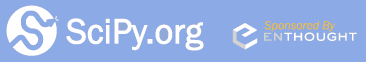## Sunday, October 11, 2009

### EULER'S GAMMA !

EULER'S GAMMA

Once again ! .... we meet Leonhard Euler ... a constant named after him. Euler-Mascheroni constant which runs as .... 0.57721 … called 'gamma' ,denoted by the Greek alphabet 'gamma' and is one of the important constants of mathematics.

From an abinitio, 'gamma' is defined as;IN SCIPY

Trying it out in scipy
yields a very accurate gamma.....

Should gamma be build into scipy as pi and e ?Fig 2. pi and e in scipy

IN MATLAB

Trying it in MATLABMATLAB recognises the integral as a special integral ! ...... with a vpa, the value is obtained.

Gamma and other mathematical constants should be build into Scipy and Scipy should be intelligent enough to identify these expressions and integrals.REFERENCES